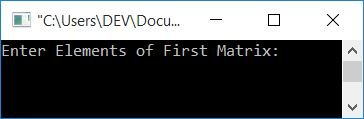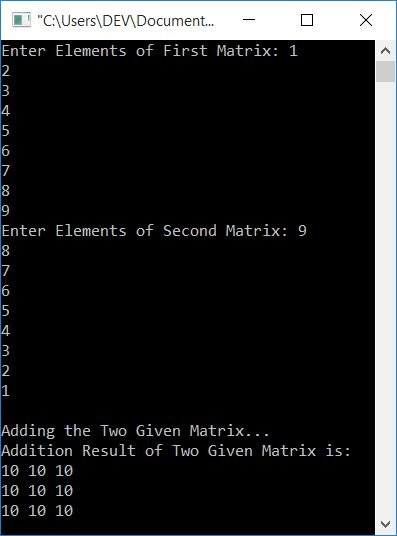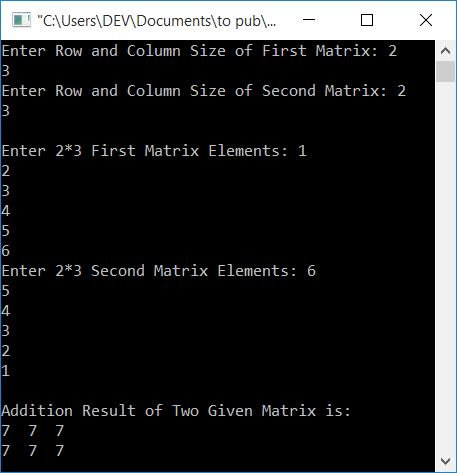# C++ Program to Add Two Matrices

In both cases, the elements of the matrix must be entered by the user at run-time.

### How can two matrices be added?

The separate article was created for matrix addition. If you're not aware of it, then I recommend that you follow the article for a complete understanding of it in a very short time.

But for now, let me tell you about it, i.e., to add two matrices, we have to add corresponding elements. That is, the first row and first column elements of the first matrix are added to the first row and first column elements of the second matrix.In a similar way, the elements in the first row and second column of the first matrix get added to the elements in the first row and second column of the second matrix. and so on.

To add two matrices in C++ programming, you have to ask the user to enter the elements of both matrices. Now add the same positioned elements to form a new matrix. After adding two matrices, display the third matrix, which is the addition result of two matrices, as shown in the following program.

## Addition of Two 3*3 Matrices in C++

The user is prompted to enter any 3*3 two-dimensional matrix. This means that the user can enter nine elements for the first matrix and nine elements for the second matrix. And then add them up to print the third matrix that will be the addition result of the given two:

```#include<iostream>
using namespace std;
int main()
{
int mat1, mat2, i, j, mat3;
cout<<"Enter Elements of First Matrix: ";
for(i=0; i<3; i++)
{
for(j=0; j<3; j++)
cin>>mat1[i][j];
}
cout<<"Enter Elements of Second Matrix: ";
for(i=0; i<3; i++)
{
for(j=0; j<3; j++)
cin>>mat2[i][j];
}
for(i=0; i<3; i++)
{
for(j=0; j<3; j++)
mat3[i][j] = mat1[i][j]+mat2[i][j];
}
cout<<"Addition Result of Two Given Matrix is:\n";
for(i=0; i<3; i++)
{
for(j=0; j<3; j++)
cout<<mat3[i][j]<<" ";
cout<<endl;
}
return 0;
}```

This program was built and runs under the Code::Blocks IDE. Here is its sample run:Now enter any nine numbers, say 1, 2, 3, 4, 5, 6, 7, 8, 9, as the elements of the first matrix, and then again nine numbers, say 9, 8, 7, 6, 5, 4, 3, 2, 1, as the elements of the second matrix. Press the ENTER key to see the addition result of two given matrices, as shown in the output given below:When a user enters the first nine elements of the matrix, they are saved in such a way that:

• The first element gets stored at mat1
• The second element gets stored at mat1
• The third element gets stored at mat1
• The fourth element gets stored at mat1
• The fifth element gets stored at mat1
• and so on.

Similarly, nine elements of the second matrix also get stored in a similar way. And the addition of two matrices goes in such a way that:

• mat3 = mat1+mat2. That is, the first element of the first matrix gets added with the first element of the second matrix and initialized as the first element of the third matrix.
• mat3 = mat1+mat2. To put it another way, the second element of both matrices is added and initialized as the second matrix of the third matrix.
• In a similar way, the matrix gets added.

Print the third matrix, which is the sum of the first two.

### Allow the user to define the size

This program allows the user to define the size of both matrices.

It should be noted that in order to define the addition of two matrices, both matrices must be of the same order. Here, the same order means the row size of the first matrix must be equal to the row size of the second matrix, and the column size of the first matrix must also be equal to the column size of the second matrix.

```#include<iostream>
using namespace std;
int main()
{
int rowOne, colOne, rowTwo, colTwo, i, j;
int mat1, mat2, sum=0;
cout<<"Enter Row and Column Size of First Matrix: ";
cin>>rowOne>>colOne;
cout<<"Enter Row and Column Size of Second Matrix: ";
cin>>rowTwo>>colTwo;
if(rowOne==rowTwo && colOne==colTwo)
{
cout<<"\nEnter "<<rowOne<<"*"<<colOne<<" First Matrix Elements: ";
for(i=0; i<rowOne; i++)
{
for(j=0; j<colOne; j++)
cin>>mat1[i][j];
}
cout<<"Enter "<<rowOne<<"*"<<colOne<<" Second Matrix Elements: ";
for(i=0; i<rowOne; i++)
{
for(j=0; j<colOne; j++)
cin>>mat2[i][j];
}
cout<<"\nAddition Result of Two Given Matrix is:\n";
for(i=0; i<rowOne; i++)
{
for(j=0; j<colOne; j++)
{
sum = mat1[i][j]+mat2[i][j];
cout<<sum<<"  ";
}
cout<<endl;
}
}
else
cout<<"\nSize Mismatched!\n";
return 0;
}```

Here is its sample run, supposing that the user has entered the row size and column size for both the matrix as 2 and 3, and that its elements for the first matrix are 1, 2, 3, 4, 5, 6, and for the second matrix are 6, 5, 4, 3, 2, 1:#### The same program in different languages

C++ Quiz

« Previous Program Next Program »